## Lemniscate Function

The lemniscate functions arise in rectifying the Arc Length of the Lemniscate. The lemniscate functions were first studied by Jakob Bernoulliand G. Fagnano. A historical account is given by Ayoub (1984), and an extensive discussion by Siegel (1969). The lemniscate functions were the first functions defined by inversion of an integral, which was first done by Gauß.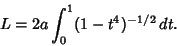(1)

Define the functions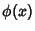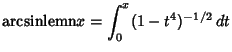(2)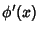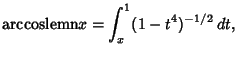(3)

where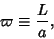(4)

and write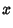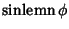(5)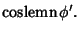(6)

There is an identity connecting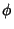and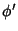since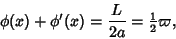(7)

so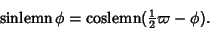(8)

These functions can be written in terms of Jacobi Elliptic Functions,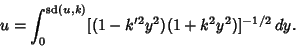(9)

Now, if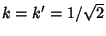, then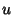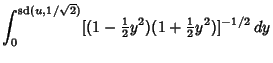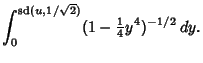(10)

Let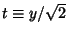so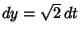,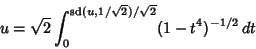(11)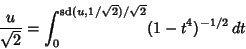(12)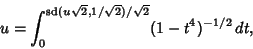(13)

and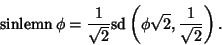(14)

Similarly,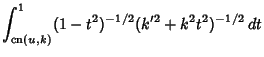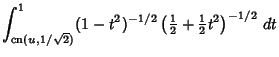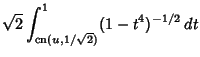(15)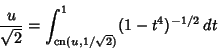(16)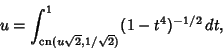(17)

and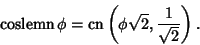(18)

We know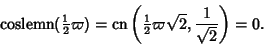(19)

But it is true that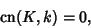(20)

so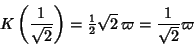(21)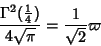(22)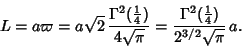(23)

By expanding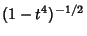in a Binomial Series and integrating term by term, the arcsinlemn function can be written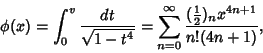(24)

where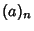is the Rising Factorial (Berndt 1994). Ramanujangave the following inversion Formula for. If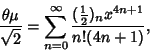(25)

where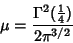(26)

is the constant obtained by letting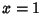and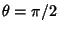, and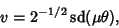(27)

then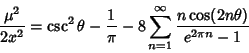(28)

(Berndt 1994). Ramanujanalso showed that if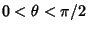, then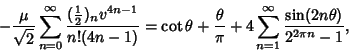(29)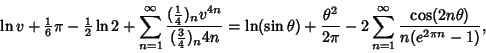(30)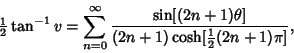(31)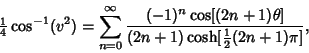(32)

and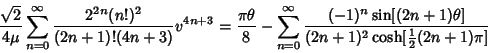(33)

(Berndt 1994).

A generalized version of the lemniscate function can be defined by letting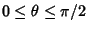and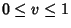. Write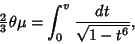(34)

where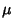is the constant obtained by settingand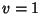. Then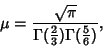(35)

and Ramanujanshowed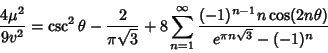(36)

(Berndt 1994).

References

Ayoub, R. The Lemniscate and Fagnano's Contributions to Elliptic Integrals.'' Arch. Hist. Exact Sci. 29, 131-149, 1984.

Berndt, B. C. Ramanujan's Notebooks, Part IV. New York: Springer-Verlag, pp. 245, and 247-255, 258-260, 1994.

Siegel, C. L. Topics in Complex Function Theory, Vol. 1. New York: Wiley, 1969.